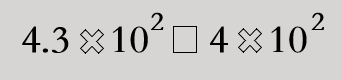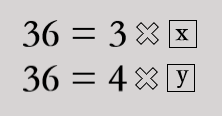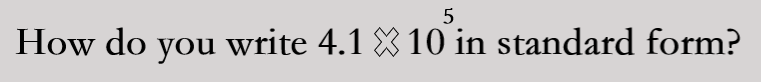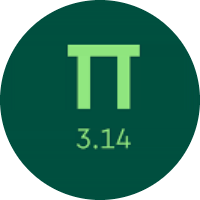# Learning the Number Theory

In this quiz, you will get to know about the interesting number theory. You will learn about factors, prime numbers, composite numbers, factorization, factor pairs, the greatest common factor, and the least common factor.

Start Quiz

Which sign makes the statement true?<

=

>

Find all the factor pairs for 27 and find the value of x and y.x = 4, y = 6

x = 6, y = 6

x = 12, y = 94100

41000

410000

Which of the following numbers is prime? Select all that apply.

19

18

11

17

Which number is a factor of 16?

14

3

8

9

What is the prime factorization of 190?

2*5*19

10*19

2*2*5*19

What is the greatest common factor of 12 and 18?

3

6

12

What is the greatest common factor of 24, 36, and 48?

24

6

18

12

What is the least common multiple of 3 and 13?

3

39

1

33

What is the least common multiple of 4, 6, 8, and 9?

72

24

108

48

Quiz/Test Summary
Title: Learning the Number Theory
Questions: 10
Contributed by: